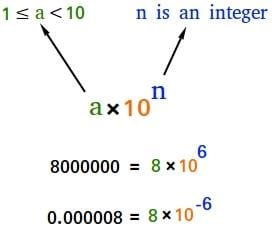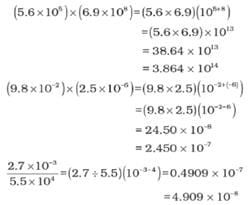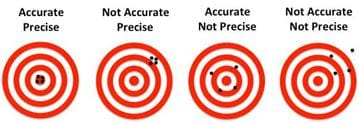NEET  >  Scientific Notation, Precision & Accuracy

# Scientific Notation, Precision & Accuracy Notes | Study Additional Documents & Tests for NEET - NEET

## Document Description: Scientific Notation, Precision & Accuracy for NEET 2022 is part of Additional Documents & Tests for NEET preparation. The notes and questions for Scientific Notation, Precision & Accuracy have been prepared according to the NEET exam syllabus. Information about Scientific Notation, Precision & Accuracy covers topics like and Scientific Notation, Precision & Accuracy Example, for NEET 2022 Exam. Find important definitions, questions, notes, meanings, examples, exercises and tests below for Scientific Notation, Precision & Accuracy.

Introduction of Scientific Notation, Precision & Accuracy in English is available as part of our Additional Documents & Tests for NEET for NEET & Scientific Notation, Precision & Accuracy in Hindi for Additional Documents & Tests for NEET course. Download more important topics related with notes, lectures and mock test series for NEET Exam by signing up for free. NEET: Scientific Notation, Precision & Accuracy Notes | Study Additional Documents & Tests for NEET - NEET
 1 Crore+ students have signed up on EduRev. Have you?

UNCERTAINTY IN MEASUREMENT
Many a times in the study of chemistry, one has to deal with experimental data as well as theoretical calculations. There are meaningful ways to handle the numbers conveniently and present the data realistically with certainty to the extent possible.
1. Scientific Notation
2. Significant Figures
3. Dimension Analysis

1. Scientific Notation
Scientific Notation is a way of expressing numbers that are too big or too small to be conveniently written in decimal form.
In which any number can be represented in the form N × 10n
(Where n is an exponent having positive or negative values and N can vary between 1 to 10).Scientific notation

Example

• We can write 232.508 as 2.32508 × 102 in scientific notation. Similarly, 0.00016 can be written as 1.6 × 10–4
• Thus, we can write 232.508 as 2.32508 × 102 in scientific notation. Note that while writing it, the decimal had to be moved to the left by two places and same is the exponent (2) of 10 in the scientific notation.
• Similarly, 0.00016 can be written as 1.6 × 10–4. Here the decimal has to be moved four places to the right and (– 4) is the exponent in the scientific notation.

MULTIPLICATION AND DIVISION FOR EXPONENTIAL NUMBERS
These two operations follow the same rules which are there for exponential numbers, i.e.ADDITION AND SUBTRACTION FOR EXPONENTIAL NUMBERS
For these two operations, first the numbers are written in such a way that they have the same exponent. After that, the coefficients (digit terms) are added or subtracted as the case may be.
Thus, for adding 6.65 × 104 and 8.95 × 103, exponent is made same for both the numbers.
Thus, we get (6.65 × 104) + (0.895 × 104)

PRECISION AND ACCURACY
Every experimental measurement has some amount of uncertainty associated with it. However, one would always like the results to be precise and accurate. Precision and accuracy are often referred to while we talk about the measurement.
Precision and accuracy are often referred to while we talk about the measurement.

Accuracy refers to the closeness of a measured value to a standard or known value
Example: if in lab you obtain a weight measurement of 3.2 kg for a given substance, but the actual or known weight is 10 kg, then your measurement is not accurate. In this case, your measurement is not close to the known value.

Precision refers to the closeness of two or more measurements to each other.
Using the example above, if you weigh a given substance five times, and get 3.2 kg each time, then your measurement is very precise. Precision is independent of accuracy. You can be very precise but inaccurate, as described above. You can also be accurate but imprecise.
Example: If on average, your measurements for a given substance are close to the known value, but the measurements are far from each other, then you have accuracy without precision.

Example
If the true value for a result is 2.00 g.
(a) Student ‘A’ takes two measurements and reports the results as 1.95 g and 1.93 g.

• These values are precise as they are close to each other but are not accurate.

(b) Another student repeats the experiment and obtains 1.94 g and 2.05 g as the results for two measurements.

• These observations are neither precise nor accurate.

(c) When a third student repeats these measurements and reports 2.01g and 1.99 g as the result.

• These values are both precise and accurate.Examples:
Q.1. Which of the following options is not correct?
(a) 8008 = 8.008 x 103
(b) 208 = 3
(c) 5000 = 5.0 x 103
(d) 2.0034 = 4
Ans: (d)
Solution:
2.0034 = 4

Q.2. Exponential notation in which any number can be represented in the form, Nx 10here N is termed as
(a) non –digit term
(b) digit term
(c) numeral
(d) base term

Ans: (b)
Solution:
In exponential notation N × 10n, N is a number called digit term which varies between 1.000 and 9.000….

Q.3. In scientific notation,0.00016 can be written as
(a) 1.6 x 10-4
(b) 1.6 x 10-3
(c) 1.6 x 10-2
(d)  1.6 x 10-1
Ans: (a)
Solution:
0.00016 can be written as 1.6 × 10-4  in scientific notation

4. Addition of 6.65 x 104 and 8.95 x 103, in terms of scientific notation will be
(a) 7.545  104
(b) 75.45  10 3
(c) 754.5  102
(d) 75.45  100
Ans: (a)
Solution:
6.65 × 104 + 8.95 × 103
= (6.65 + 0.895) × 104 = 7.545 × 104

Q.5. The substraction of two numbers
2.5 x 10-2 -4.8 x 10 -3 gives the following value.
(a) 2.02 x 10-3
(b) 2.02 x 10-2
(c) 2.02 x 10-1
(d) 2.02 x 100
Ans: (b)
Solution:
2.5 × 10-2 - 4.8 × 10-3
= 2.5 × 10-2 — (0.48 × 10-2) = 2.02 × 10-2

Q.6. A refers to the closeness of various measurements for the same quantity. B is the agreement of a particular value to the true value of the result. A and B respective are
(a) A  → Significant figures, B → accuracy
(b) A → accuracy, B → precision
(c) A → Precision, B → accuracy
(d) A → significant figures,  → precision

Ans: (c)

Q.7. Which of the following statement is/are true?
(a) Every experimental measurement has zero amount of uncertainty associated with it
(b) One would always like the result to be precise and accurate
(c) Precision and accuracy are often referred to while we talk about the measurement
(d) Both (b) and (c)
Ans:
(d)
Solution:
Every experimental measurement has some amount of uncertainty associated with it.

Try Yourself!

Question for Scientific Notation, Precision & Accuracy
Try yourself:Which of the following statement is correct ?

Question for Scientific Notation, Precision & Accuracy
Try yourself:Two students X and Y report the weight of the same substance as 5.0g and 5.00g  respectively. Which of the following statements is correct ?

The document Scientific Notation, Precision & Accuracy Notes | Study Additional Documents & Tests for NEET - NEET is a part of the NEET Course Additional Documents & Tests for NEET.
All you need of NEET at this link: NEET

## Additional Documents & Tests for NEET

10 videos|153 docs|55 tests
 Use Code STAYHOME200 and get INR 200 additional OFF

## Additional Documents & Tests for NEET

10 videos|153 docs|55 tests

### How to Prepare for NEET

Read our guide to prepare for NEET which is created by Toppers & the best Teachers

Track your progress, build streaks, highlight & save important lessons and more!

,

,

,

,

,

,

,

,

,

,

,

,

,

,

,

,

,

,

,

,

,

,

,

,

;